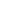# Free CBSE Class 6 Maths Worksheets

Download free printable Maths Worksheets to practice. With thousands of questions available, you can generate as many Maths Worksheets as you want.

## Sample CBSE Class 6 Maths Worksheet Questions

1.

How many integers are there between -8 and 2

1.

7

2.

4

3.

0

4.

9

2.

Which integer is greater than -5

1.

-7

2.

-9

3.

-7

4.

-1

3.

Combine terms: 12a + 26b -4b – 16a.

1.

4a + 22b

2.

-28a+30b

3.

-4a+22b

4.

28a+30b

4.

Roman number D represents

1.

100

2.

50

3.

500

4.

1000

5.

How many sides a decagon have?

1.

10

2.

5

3.

7

4.

8

6.

The sum of two number is 55 and the H.C.F and L.C.M of these numbers are 5 and 120 respectively. Find the sum of the reciprocals of the numbers.

1.

11/24

2.

10/26

7.

evaluate the difference between the place values of two 9's in the number 79520986.

1.

8999910

2.

88991000

3.

8999100

8.

The greatest number that will divide 10 and 18 is

1.

4

2.

2

3.

5

4.

3

9.

12 × (45 + 30) =
1.
A.
(12
×
45) + (12
×
30)
2.
B.
12
×
65
3.
C.
12
×
45
×
30
4.
D.
(12
×
45)
×
(12
×
30)
10.

The parking lot of a fairground has a capacity of 1560 cars. On Tuesday, the ratio of the empty parking spots to occupied parking spots is 19:33. The number of cars that were parked there on that day is:

1.

1024

2.

570

3.

990

## Find more Maths Worksheets

Worksheets by UrbanPro

Our worksheets are designed to help students explore various topics, practice skills and enrich their subject knowledge, to improve their academic performance. Designed by Experts who have extensive experience and expertise in teaching a subject, these worksheets will improve your child's problem-solving skills and subject knowledge in a fun and interactive manner.
Check out our free customized worksheets across school boards, grades, subjects and levels of subject knowledge. You can download, print and share these worksheets with anyone, anywhere, anytime!

Get a custom worksheet to practice!

Select your topic & see the magic.

subjectSelect Chapter(s)

Chapters & Subtopics